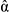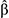The correlation between regression coefficients: combined significance testing for calibration and quantitation of bias

Abstract

In the analytical sciences regression methods have two main uses – in calibrations in instrumental analysis, and in testing for bias in method comparison studies. In first order (straight line) regression the true values of the intercept α and the slope β are independent of each other but their estimated valuesandare not independent. This can be appreciated visually by considering the straight lines joining all the individual pairs of points; those with a large slope will have a small intercept and vice versa, so the correlation betweenandis negative and possibly substantial. This correlation has important consequences when the estimated coefficients are used for significance testing in the interpretation of the regression line.Publication details

The article was received on 05 Mar 2019, accepted on 05 Mar 2019 and first published on 27 Mar 2019

Article type: AMC Technical Brief
DOI: 10.1039/C9AY90041A
Anal. Methods, 2019,11, 1845-1848

•The correlation between regression coefficients: combined significance testing for calibration and quantitation of bias

Analytical Methods Committee AMCTB No 87, Anal. Methods, 2019, 11, 1845
DOI: 10.1039/C9AY90041A